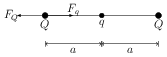# Coulomb's Law

The force $\vec{F}$ between two charges $q_1$ and $q_2$ placed in free space and separated by a distance $r$ is given by \begin{align} \vec{F}=\frac{1}{4\pi\epsilon_0}\frac{q_1 q_2}{r^2}\hat{r} \end{align} where $\epsilon_0$ is the permittivity of free space. The force is repulsive if charges are of the same type and it is attractive if charges are of different type.

## Problems from IIT JEE

Problem (IIT JEE 1987): A charge $q$ is placed at the centre of the line joining two equal charges $Q$. The system of the three charges will be in equilibrium if $q$ is equal to,

1. $-Q/2$
2. $-Q/4$
3. $+Q/4$
4. $+Q/2$

Solution:Let the separation between two particles of charges $Q$ be $2a$. Coulomb's forces on the charge $q$ due to other two charges are equal and opposite. Hence, charge $q$ is always at equilibrium irrespective of its sign and magnitude. Coulomb's force on charge $Q$ due to another charge $Q$ is repulsive in nature and has magnitude $F_Q=Q^2/(16\pi\epsilon_0 a^2)$. For the charge $Q$ to be in equilibrium, Coulomb's force on it due to charge $q$ should be attractive and of magnitude $F_Q$ i.e., \begin{align} Q^2/(16\pi\epsilon_0 a^2)=-{Qq}/{(4\pi\epsilon_0 a^2)}, \end{align} which gives $q=-Q/4$.